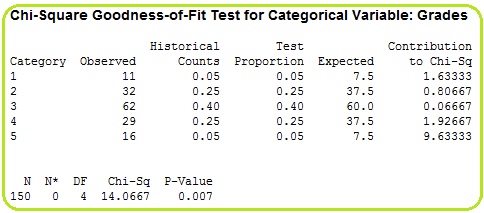Problem

# Xr15-10 Grades assigned by an economics instructor have historically followed a symmetrica...

Xr15-10 Grades assigned by an economics instructor have historically followed a symmetrical distribution: 5% A’s, 25% B’s, 40% C’s, 25% D’s, and 5% F’s. This year, a sample of 150 grades was drawn and the grades (1 = A, 2 = B, 3 = C, 4 = D, and 5 = F) were recorded. Can you conclude, at the 10% level of significance, that this year’s grades are distributed differently from grades in the past?

#### Step-by-Step Solution

Solution 1

Grades are assigned by an economics instructor have historically followed a symmetrical distribution.

The claim is that the grades are distributed differently from the grades in the past From the given data we have, the null and alternative hypotheses are $$H_{0}: p_{1}=.05, p_{2}=.25, p_{3}=.40, p_{4}=.25, p_{5}=0.05$$

$$H_{1}:$$ At least one $$p_{i}$$ is not equal to its specified value

The test statistic for the Chi-Square goodness-of-fit $$\left(\chi^{2}\right)$$ is given by,

$$\chi^{2}=\sum_{i=1}^{k} \frac{\left(f_{i}-e_{i}\right)^{2}}{e_{i}}$$

Where,

$$e_{i}=$$ expected frequencies.

$$f_{i}=$$ observed frequencies.

Given level of significance $$\alpha=.10$$

The following are the steps to get the  χ2 - statistic for the Account receivable data using MINITAB.

1) Click Start, Table, and Chi-square Goodness-of-fit Test (One Variable)….

2) Type the observed values into the Categorical data and specify the variable name.

3) Click Proportions specified by historical counts and Input constants type the values of the proportions under the null hypothesis(0.05,0.25,0.40,0.25, 0.05)

4) Click OK.

We get the following output,From the above work,

The test statistic, χ2 =14.0667

The P-value is 0.007.

Here we observe that the P-value is less than the significance level 0.10, so we reject the null hypothesis. Therefore, there is enough evidence to support the claim that the grades are distributed differently from the steps in the past.